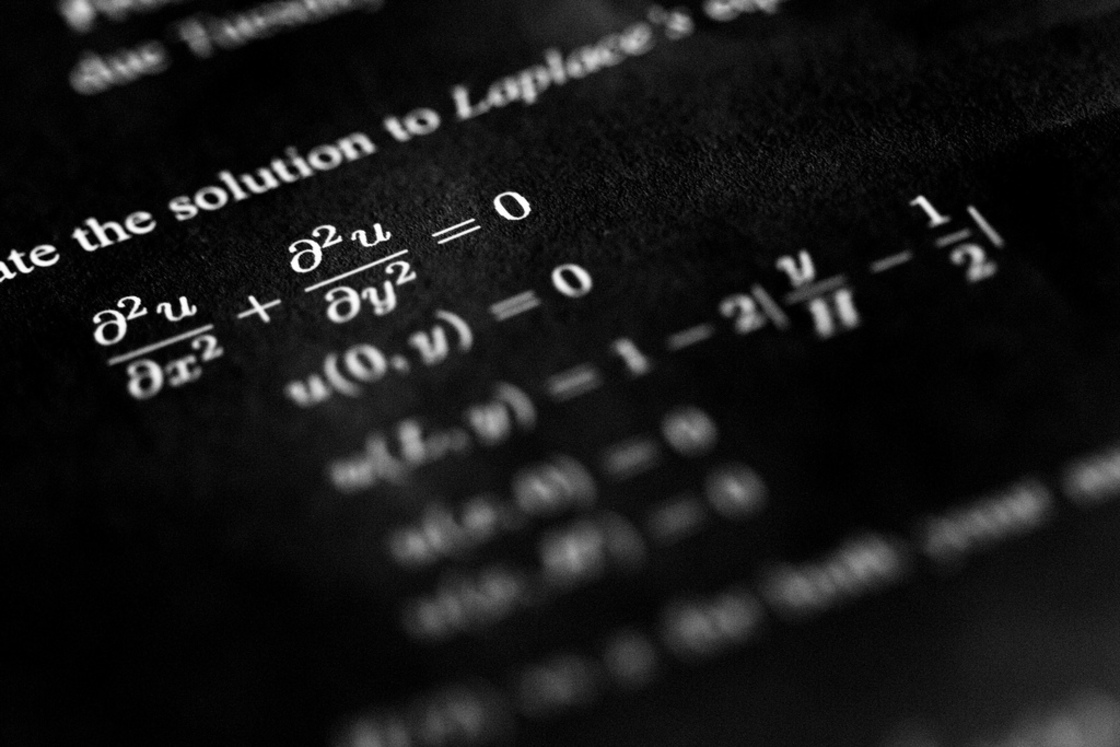Mar 1 1 – 10 March 2011 at the Chinese University of Hong Kong

# Nonlinear Partial Differential Equations: recent advances and future directions

This Institue will provide a forum for the dissemination of current advances in the field of nonlinear partial differential equations, and related topics including differential geometry, mathematical biology, fluid mechanics, etc
The focus will be on the interaction of experts in diverse directions working on nonlinear partial differential equations, and other applied sciences. Our primary goal is to expose a limited number of postdoctoral fellows and advanced graduate students to current problems associated with nonlinear partial differential equations, and to highlight some of the recent mathematical advances in Navier-Stokes equations, nonlinear diffusion and kinetic equations, Einstein vacuum equations, De Giorgi conjecture, spikes and higher dimensional concentration phenomena, reaction-diffusion systems and localized behavior in mathematical biology, etc.